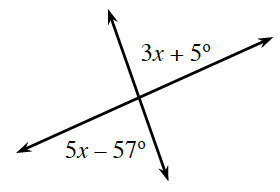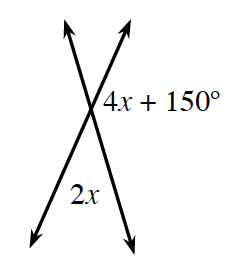### Home > INT2 > Chapter 1 > Lesson 1.3.1 > Problem1-77

1-77.

Examine the diagrams below. What is the angle pair relationship between the labeled angles? Use the relationship to write an equation and solve for $x$. Then confirm your answer by calculating the angle measures of the labeled angles.

1.They are vertical angles and the measures are congruent.

$3x+5^\circ=5x−57^\circ$
$x=31^\circ$

1.They form a straight angle pair, so they are supplementary.